# Missing values in Moving Aggregation vs Moving Average

…and can someone explain to me how the “Moving Average” node handles missing values?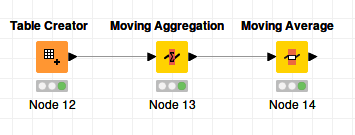Moving Aggregation config: length: 2, type: forward.
Moving Average config: length: 2, type: forward simple.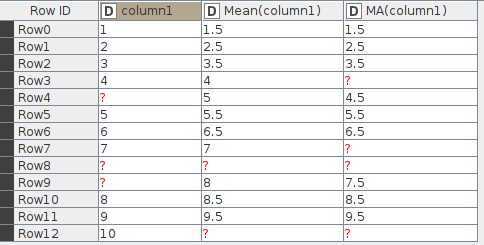Take a look at the description of the node.
br

I am often guilty of scanning the help text too fast, but…

The only vaguely relevant part of the help text is this: “For all window based methods … the cells that do not have a complete window (at the beginning and the end of the table) are filled with Missing Values.”

Where the missing value at the end of the table comes from is obvious. This is identical for Moving Aggregation and the Moving Average nodes. It makes sense.

I was referring to the missing value in the middle of the Moving Average node results. The Moving Average over [4, ?] is [?]… The moving Average over [?, 5] is [4.5]. Weird.

The Moving Average over [7, ?] is [?]. The Moving Average over [?, ?] is [?]. So far so good. The Moving Average over [?, 8] is [7.5]… The window somehow looks back to the last non-missing value before the missing value.

Let’s try a window of size 3.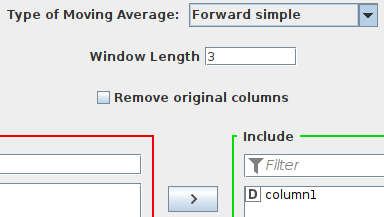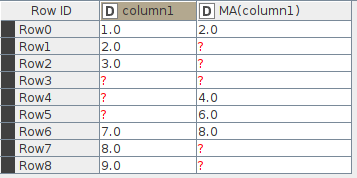Check out Row4. The moving average should be over [?,?,7]. But the result is somehow 4.0. Obviously the moving average is over [2.0, 3.0, 7.0]. As a result of the missing values the moving average somehow looks back 3 and 2 rows.

I simply don’t get it.

Here’s what I think is going on.

The mean aggregation in the Moving Aggregation node doesn’t allow for missing values. It calculates the mean based on the number of values actually present. So with a window size of three, this looks like:As you can see here, the forward moving average is calculated as
`Forward MA = sum of the values in the window / amount of values in the window`

On the other hand, the moving average node is different. The forward simple moving average doesn’t like the final value in the window to be missing, so it would return missing for those rows. That takes care of the 3-row windows starting on Row1, Row2, Row3, Row7, and Row8.

After checking the last value in the window, if there are missing values at the start of the window, then the node disregards those rows. They no longer exist; this is the case for Row3, Row4, and Row5

Once all of this is done, it finally calculates the forward simple average of the nth row as:
`1/windowSize * sum{value_n, ... , value_n+windowSize-1}`

So for Row0, the resulting value is 2.
Row6 is treated as if it were Row3, and the resulting value is 6.If you remove the rows with missing values before using the Moving Average node, you get basically the same result, without all the intervening missing value question marks in either column.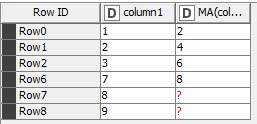3 Likes

Hmmm… okay. Still, the behavior is a bit funny.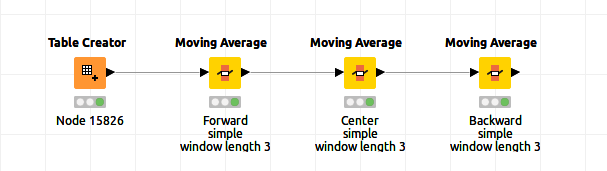Result: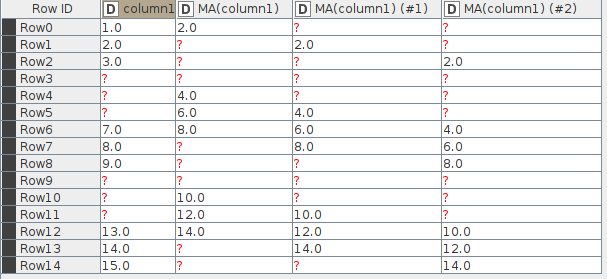The gap in the data travels up even for “Center Simple” with a window length of 3, while the centers of the data chunks (the numbers 2, 8 and 14 in this case) stay put.

This topic was automatically closed 182 days after the last reply. New replies are no longer allowed.